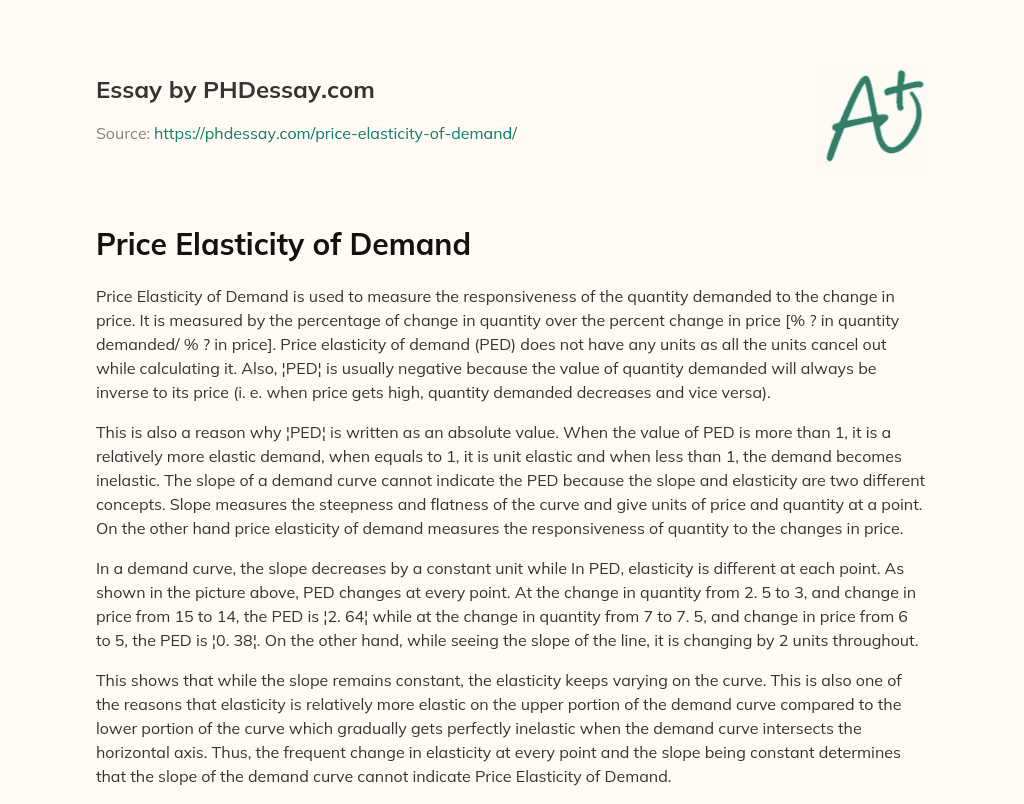Last Updated 17 Jan 2020

# Price Elasticity of Demand

Category Microeconomics
Words 372 (1 page)
9 views

Price Elasticity of Demand is used to measure the responsiveness of the quantity demanded to the change in price. It is measured by the percentage of change in quantity over the percent change in price [% ? in quantity demanded/ % ? in price]. Price elasticity of demand (PED) does not have any units as all the units cancel out while calculating it. Also, ¦PED¦ is usually negative because the value of quantity demanded will always be inverse to its price (i. e. when price gets high, quantity demanded decreases and vice versa).

This is also a reason why ¦PED¦ is written as an absolute value. When the value of PED is more than 1, it is a relatively more elastic demand, when equals to 1, it is unit elastic and when less than 1, the demand becomes inelastic. The slope of a demand curve cannot indicate the PED because the slope and elasticity are two different concepts. Slope measures the steepness and flatness of the curve and give units of price and quantity at a point. On the other hand price elasticity of demand measures the responsiveness of quantity to the changes in price.

In a demand curve, the slope decreases by a constant unit while In PED, elasticity is different at each point. As shown in the picture above, PED changes at every point. At the change in quantity from 2. 5 to 3, and change in price from 15 to 14, the PED is ¦2. 64¦ while at the change in quantity from 7 to 7. 5, and change in price from 6 to 5, the PED is ¦0. 38¦. On the other hand, while seeing the slope of the line, it is changing by 2 units throughout.

Order custom essay Price Elasticity of Demand with free plagiarism report

GET ORIGINAL PAPER

This shows that while the slope remains constant, the elasticity keeps varying on the curve. This is also one of the reasons that elasticity is relatively more elastic on the upper portion of the demand curve compared to the lower portion of the curve which gradually gets perfectly inelastic when the demand curve intersects the horizontal axis. Thus, the frequent change in elasticity at every point and the slope being constant determines that the slope of the demand curve cannot indicate Price Elasticity of Demand.This essay was written by a fellow student. You can use it as an example when writing your own essay or use it as a source, but you need cite it.

## Get professional help and free up your time for more important courses

Starting from 3 hours delivery 450+ experts on 30 subjects
get essay help 124  experts online

Did you know that we have over 70,000 essays on 3,000 topics in our database?